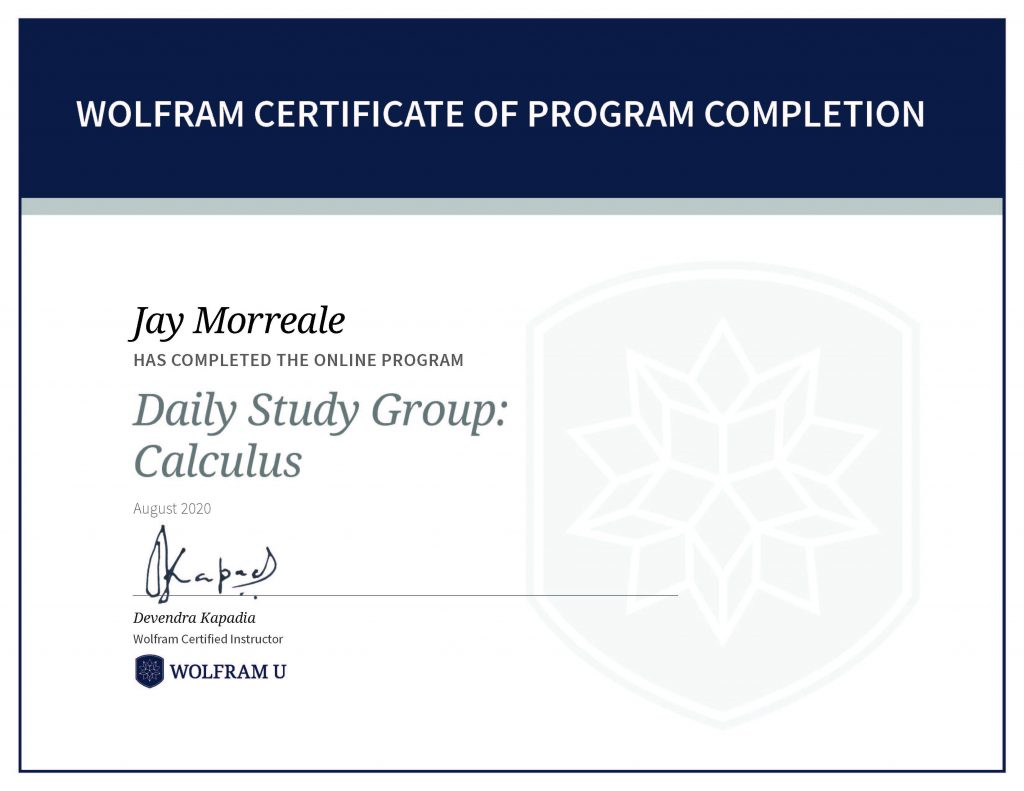# Wolfram U Daily Study Group: Calculus

The three week Calculus Study Group (4) uses the Introduction to Calculus self study course as part of the daily lectures and covers functions, limits, differential calculus, and integral calculus. This course is a good way to prepare for AP Calculus and is a great refresher on the topic.

The functions 3x, the derivative of 3x, ex, the derivative of ex, and 2x, the derivative of 2x are shown in the plot on the left in the figure below to show the awesome properties of ex, for example. The derivative of ex is ex so the slope anywhere on the line is just the value of the function at that point.

The tangent line and normal line are shown in the plot on the right for ex=3. This point was chosen as an example. The functions used to create the tangent and normal lines are as follows.

• f(x)=ex
• Tangent(x)=f′(x)(x−x0)+f(x0) at x0=3
• Normal(x)=(−1/f′(x))(x−x0)+f(x0) at x0=3

The area under ex to x0=3 can be found by integrating the curve from −∞ to 3 and is e3. Thus, the area under the curve is also the value of the function at that point.

To receive a program completion certificate you must pass three quizzes.Wolfram Certificate of Program Completion, Jay Morreale has completed the online program Daily Study Group: Calculus.

# Wolfram U Introduction to Notebooks

The three week Introduction to Notebooks Study Group (3) covered notebooks, machine learning, and image processing. Daily study group lectures included content from Wolfram courses and live instructor instruction that varied from week to week and included:

• Week 1: Introduction to Notebooks covered styling, formatting and creating interactive documents.
• Week 2: Machine Learning covered classification, regression, cluster analysis and anomaly detection.
• Week 3: Image processing covered digital image processing, image representation, and classical operations on images.

I used the image processing tools to find clusters in a photograph of an Arduino MKR WiFi 1010 board, colorized the clusters, and add colorized text to make a composite illustration that demonstrates the use of these features.

To receive a course completion certificate you must pass three quizzes.

# Wolfram Technology Level 1 Certificate

I received my Wolfram Technology Level 1 Certificate for demonstrating proficiency in the Wolfram Language Programming Fundamentals! To receive the Level 1 certificate, students needed to complete and pass a 20 question exercise. Each question required you to write a program to solve a problem described in the question. Most questions gave hints on what functions should be used to solve the problem.

So, now it get interesting because the exercises run on the Wolfram cloud and the answers are graded by an auto-grader. Basically, we need to convince a bot that we can program. There are multiple correct ways to solve the problem, but the auto-grader doesn’t like just any solution so it might take 3 or more program submission to get a pass on a question. The auto-grader seems to prefer efficient code so you can have the correct from of the program, but auto-grader will reject it until you submit the more or most efficient answer.

Some questions specified the use of random integers or random selections. The auto-grader did not seem to be able to accommodate program answers that produce random outputs. Several other questions seemed to give the auto-grader problems, and it’s unclear why. The answers to these seven questions were submitted to the study group staff by email and graded by hand.